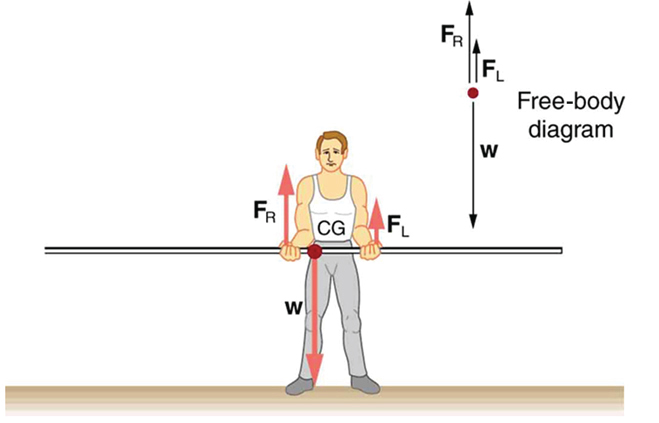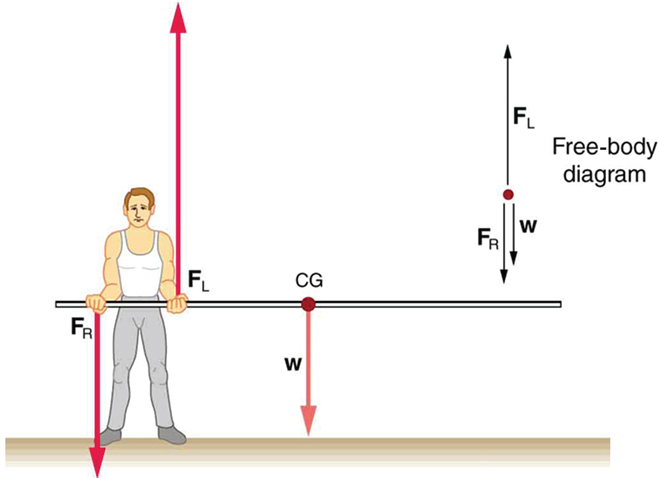# 9.4 Applications of statics, including problem-solving strategies  (Page 2/3)

 Page 2 / 3

Similar observations can be made using a meter stick held at different locations along its length.A pole vaulter is holding a pole horizontally with both hands. The center of gravity is near his right hand.A pole vaulter is holding a pole horizontally with both hands. The center of gravity is to the left side of the vaulter.

If the pole vaulter holds the pole as shown in [link] , the situation is not as simple. The total force he exerts is still equal to the weight of the pole, but it is not evenly divided between his hands. (If ${F}_{L}={F}_{R}$ , then the torques about the cg would not be equal since the lever arms are different.) Logically, the right hand should support more weight, since it is closer to the cg. In fact, if the right hand is moved directly under the cg, it will support all the weight. This situation is exactly analogous to two people carrying a load; the one closer to the cg carries more of its weight. Finding the forces ${F}_{L}$ and ${F}_{R}$ is straightforward, as the next example shows.

If the pole vaulter holds the pole from near the end of the pole ( [link] ), the direction of the force applied by the right hand of the vaulter reverses its direction.

## What force is needed to support a weight held near its cg?

For the situation shown in [link] , calculate: (a) ${F}_{R}$ , the force exerted by the right hand, and (b) ${F}_{L}$ , the force exerted by the left hand. The hands are 0.900 m apart, and the cg of the pole is 0.600 m from the left hand.

Strategy

[link] includes a free body diagram for the pole, the system of interest. There is not enough information to use the first condition for equilibrium $\text{(net}\phantom{\rule{0.25em}{0ex}}F=0$ ), since two of the three forces are unknown and the hand forces cannot be assumed to be equal in this case. There is enough information to use the second condition for equilibrium $\text{(net}\phantom{\rule{0.25em}{0ex}}\tau =0\text{)}$ if the pivot point is chosen to be at either hand, thereby making the torque from that hand zero. We choose to locate the pivot at the left hand in this part of the problem, to eliminate the torque from the left hand.

Solution for (a)

There are now only two nonzero torques, those from the gravitational force ( ${\tau }_{\text{w}}$ ) and from the push or pull of the right hand ( ${\tau }_{R}$ ). Stating the second condition in terms of clockwise and counterclockwise torques,

$\text{net}\phantom{\rule{0.25em}{0ex}}{\tau }_{\text{cw}}=\text{–net}\phantom{\rule{0.25em}{0ex}}{\tau }_{\text{ccw}}\text{.}$

or the algebraic sum of the torques is zero.

Here this is

${\tau }_{R}={\mathrm{–\tau }}_{\text{w}}$

since the weight of the pole creates a counterclockwise torque and the right hand counters with a clockwise torque. Using the definition of torque, $\tau =\text{rF}\phantom{\rule{0.25em}{0ex}}\text{sin}\phantom{\rule{0.25em}{0ex}}\theta$ , noting that $\theta =90º$ , and substituting known values, we obtain

$\left(0\text{.}\text{900 m}\right)\left({F}_{R}\right)=\left(0\text{.600 m}\right)\left(\mathit{mg}\right)\text{.}$

Thus,

$\begin{array}{lll}{F}_{R}& =& \left(0.667\right)\left(\text{5.00 kg}\right)\left(9.80\phantom{\rule{0.25em}{0ex}}{\text{m/s}}^{2}\right)\\ & =& \text{32.7 N.}\end{array}$

Solution for (b)

The first condition for equilibrium is based on the free body diagram in the figure. This implies that by Newton’s second law:

${F}_{L}+{F}_{R}–\text{mg}=0$

From this we can conclude:

${F}_{L}+{F}_{R}=w=\text{mg}$

Solving for ${F}_{L}$ , we obtain

$\begin{array}{lll}{F}_{L}& =& \mathit{mg}-{F}_{R}\\ & =& \mathit{mg}-\text{32}\text{.}7 N\\ & =& \left(\text{5.00 kg}\right)\left(\text{9.80}\phantom{\rule{0.25em}{0ex}}{\text{m/s}}^{2}\right)-\text{32.7 N}\\ & =& \text{16.3 N}\end{array}$

Discussion

${F}_{L}$ is seen to be exactly half of ${F}_{R}$ , as we might have guessed, since ${F}_{L}$ is applied twice as far from the cg as ${F}_{R}$ .

If the pole vaulter holds the pole as he might at the start of a run, shown in [link] , the forces change again. Both are considerably greater, and one force reverses direction.

## Take-home experiment

This is an experiment to perform while standing in a bus or a train. Stand facing sideways. How do you move your body to readjust the distribution of your mass as the bus accelerates and decelerates? Now stand facing forward. How do you move your body to readjust the distribution of your mass as the bus accelerates and decelerates? Why is it easier and safer to stand facing sideways rather than forward? Note: For your safety (and those around you), make sure you are holding onto something while you carry out this activity!

## Phet explorations: balancing act

Play with objects on a teeter totter to learn about balance. Test what you've learned by trying the Balance Challenge game.

## Summary

• Statics can be applied to a variety of situations, ranging from raising a drawbridge to bad posture and back strain. We have discussed the problem-solving strategies specifically useful for statics. Statics is a special case of Newton’s laws, both the general problem-solving strategies and the special strategies for Newton’s laws, discussed in Problem-Solving Strategies , still apply.

## Conceptual questions

When visiting some countries, you may see a person balancing a load on the head. Explain why the center of mass of the load needs to be directly above the person’s neck vertebrae.

## Problems&Exercises

To get up on the roof, a person (mass 70.0 kg) places a 6.00-m aluminum ladder (mass 10.0 kg) against the house on a concrete pad with the base of the ladder 2.00 m from the house. The ladder rests against a plastic rain gutter, which we can assume to be frictionless. The center of mass of the ladder is 2 m from the bottom. The person is standing 3 m from the bottom. What are the magnitudes of the forces on the ladder at the top and bottom?

In [link] , the cg of the pole held by the pole vaulter is 2.00 m from the left hand, and the hands are 0.700 m apart. Calculate the force exerted by (a) his right hand and (b) his left hand. (c) If each hand supports half the weight of the pole in [link] , show that the second condition for equilibrium $\text{(net}\phantom{\rule{0.25em}{0ex}}{\tau }_{}=\text{0)}$ is satisfied for a pivot other than the one located at the center of gravity of the pole. Explicitly show how you follow the steps in the Problem-Solving Strategy for static equilibrium described above.

Why is the sky blue...?
It's filtered light from the 2 forms of radiation emitted from the sun. It's mainly filtered UV rays. There's a theory titled Scatter Theory that covers this topic
Mike
A heating coil of resistance 30π is connected to a 240v supply for 5min to boil a quantity of water in a vessel of heat capacity 200jk. If the initial temperature of water is 20°c and it specific heat capacity is 4200jkgk calculate the mass of water in a vessel
A thin equi convex lens is placed on a horizontal plane mirror and a pin held 20 cm vertically above the lens concise in position with its own image the space between the undersurface of d lens and the mirror is filled with water (refractive index =1•33)and then to concise with d image d pin has to
Be raised until its distance from d lens is 27cm find d radius of curvature
Azummiri
what happens when a nuclear bomb and atom bomb bomb explode add the same time near each other
A monkey throws a coconut straight upwards from a coconut tree with a velocity of 10 ms-1. The coconut tree is 30 m high. Calculate the maximum height of the coconut from the top of the coconut tree? Can someone answer my question
v2 =u2 - 2gh 02 =10x10 - 2x9.8xh h = 100 ÷ 19.6 answer = 30 - h.
Ramonyai
why is the north side is always referring to n side of magnetic
who is a nurse
A nurse is a person who takes care of the sick
Bukola
a nurse is also like an assistant to the doctor
explain me wheatstone bridge
good app
samuel
Wheatstone bridge is an instrument used to measure an unknown electrical resistance by balancing two legs of a bridge circuit, one leg of which includes the unknown component.
MUHD
Rockwell Software is Rockwell Automation’s "Retro Encabulator". Now, basically the only new principle involved is that instead of power being generated by the relative motion of conductors and fluxes, it’s produced by the modial interaction of magneto-reluctance and capacitive diractance. The origin
Chip
what refractive index
write a comprehensive note on primary colours
relationship between refractive index, angle of minimum deviation and angle of prism
Harrison
Who knows the formula for binding energy,and what each variable or notation stands for?
1. A black thermocouple measures the temperature in the chamber with black walls.if the air around the thermocouple is 200 C,the walls are at 1000 C,and the heat transfer constant is 15.compute the temperature gradient
what is the relationship between G and g
G is the u. constant, as g stands for grav, accelerate at a discreet point
Mark
Olaiya
pls explain in details
Olaiya
G is a universal constant
Mark
g stands for the gravitational acceleration point. hope this helps you.
Mark
balloon TD is at a gravitational acceleration at a specific point
Mark
I'm sorry this doesn't take dictation very well.
Mark
Can anyone explain the Hooke's law of elasticity?
extension of a spring is proportional to the force applied so long as the force applied does not exceed the springs capacity according to my textbook
Amber
does this help?
Amber
Yes, thanks
Olaiya
so any solid can be compressed how compressed is dependent upon how much force is applied F=deltaL
Amber
sorry, the equation is F=KdeltaL delta is the triangle symbol and L is length so the change in length is proportional to amount of Force applied I believe that is what Hookes law means. anyone catch any mistakes here please correct me :)
Amber
I think it is used only for solids and not liquids, isn't it?
Olaiya
basically as long as you dont exceed the elastic limit the object should return to it original form but if you exceed this limit the object will not return to original shape as it will break
Amber
Thanks for the explanation
Olaiya
yh, liquids don't apply here, that should be viscosity
Chiamaka
hope it helps 😅
Amber
also, an object doesnt have to break necessarily, but it will have a new form :)
Amber
Yes
Olaiya
yeah, I think it is for solids but maybe there is a variation for liquids? that I am not sure of
Amber
ok
Olaiya
good luck!
Amber
Same
Olaiya
aplease i need a help on spcific latent heat of vibrations
Bilgate
specific latent heat of vaporisation
Bilgate
how many kilometers makes a mile
Faizyab
Aakash
equal to 1.609344 kilometers.
MUHDByByBy